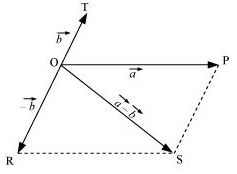## Pages

### Motion in a plane NCERT Solutions Class 11 Physics - Solved Exercise Question 4.6

Question 4.6:

Establish the following vector inequalities geometrically or otherwise :
(a) |a+b| < |a| + |b|
(b) |a+b| > | |a| −|b| |
(c) |ab| < |a| + |b|
(d) |ab| > | |a| − |b| |

Solution:

(a) Let two vectors a and b be represented by the adjacent sides of a parallelogram OMNP, as shown in the given figure.Here, we can write:
OM = | a |   ...(i)
MN = OP = | b | ....(ii)
ON = | a + b |   .....(iii)
In a triangle, each side is smaller than the sum of the other two sides.
Therefore, in ΔOMN, we have:
ON < (OM + MN)
| a + b | < | a | + | b |   ....(iv)
If the two vectors a and b act along a straight line in the same direction, then we can write:
| a + b | = | a | + | b |   ..... (v)
Combining equations (iv) and (v), we get:
| a + b | ≤ | a | + | b |

(b) Let two vectors a and b be represented by the adjacent sides of a parallelogram OMNP, as shown in the given figure.Here, we have:
| OM | = | a |
| MN | = | OP | = | b |
| ON | = | a + b |
In a triangle, each side is smaller than the sum of the other two sides.
Therefore, in ΔOMN, we have:
ON + MN > OM
ON + OM > MN
| ON | > | OM - OP |   (∵ OP = MN)
| a + b | > | | a | - | b | |      ....(iv)
If the two vectors a and b act along a straight line in the same direction, then we can write:
| a + b | = | | a | - | b | |     .....(v)
Combining equations (iv) and (v), we get:
| a + b | | | a | - | b | |

(c) Let two vectors a and b be represented by the adjacent sides of a parallelogram PORS, as shown in the given figure.Here we have:
| OR | = | PS | = | b |    ...(i)
| OP | = | a |    ....(ii)
In a triangle, each side is smaller than the sum of the other two sides. Therefore, in ΔOPS, we have:
OS < OP + PS
| a - b | < | a | + | -b |
| a - b | < | a | + | b |   ... (iii)
If the two vectors act in a straight line but in opposite directions, then we can write:
| a - b | = | a | + | b |   ... (iv)
Combining equations (iii) and (iv), we get:
| a - b | | a | + | b |

(d) Let two vectors a and b be represented by the adjacent sides of a parallelogram PORS, as shown in the given figure.The following relations can be written for the given parallelogram.
OS + PS > OP    .....(i)
OS > OP - PS   ....(ii)
| a - b | > | a | - | b |    ....(iii)
The quantity on the LHS is always positive and that on the RHS can be positive or negative. To make both quantities positive, we take modulus on both sides as:
| | a - b | | > | | a | - | b | |
| a - b | > | | a | - | b | |    ....(iv)
If the two vectors act in a straight line but in the opposite directions, then we can write:
| a - b | = | | a | - | b | |     ....(v)
Combining equations (iv) and (v), we get:
| a - b | | | a | - | b | |